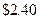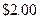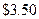Chapter 3, Problem 57P### Principles of Economics 2e

2nd Edition
Steven A. Greenlaw; David Shapiro
ISBN: 9781947172364

#### Solutions

Chapter
Section### Principles of Economics 2e

2nd Edition
Steven A. Greenlaw; David Shapiro
ISBN: 9781947172364
Textbook Problem

# A low-income county decides to set a price ceiling on bread so it can make sure that bread is affordable to the poor. Table 3.11 provides the conditions of demand and supply. What are the equilibrium price and equilibrium quantity before the price ceiling? What will the excess demand or the shortage (that is, quantity demanded minus quantity supplied) be if the price ceiling is set atAtAtTo determine

The effect of price ceiling on bread on the equilibrium price and equilibrium quantity.

Explanation

In the given question, the low-income country has decided to regulate the price ceiling so that a good (bread) can be affordable to the poor people. In the given table, the equilibrium price will be $2.80 where the quantity demanded is equal to the quantity supplied. So, the equilibrium quantity will be 7500. If the government sets the price ceiling at$2.40, then at a price of $2.40, the quantity demanded is 8,000 and the quantity supplied is 6,400. Excess demand = 8000 6400 = 1600 The excess or shortage if the price ceiling is set at$2.40 is 1600 units.

In next case, the government sets the price ceiling at $2. At a price of$2, the quantity demanded is 8500 and the quantity supplied is 5500

### Still sussing out bartleby?

Check out a sample textbook solution.

See a sample solution

#### The Solution to Your Study Problems

Bartleby provides explanations to thousands of textbook problems written by our experts, many with advanced degrees!

Get Started